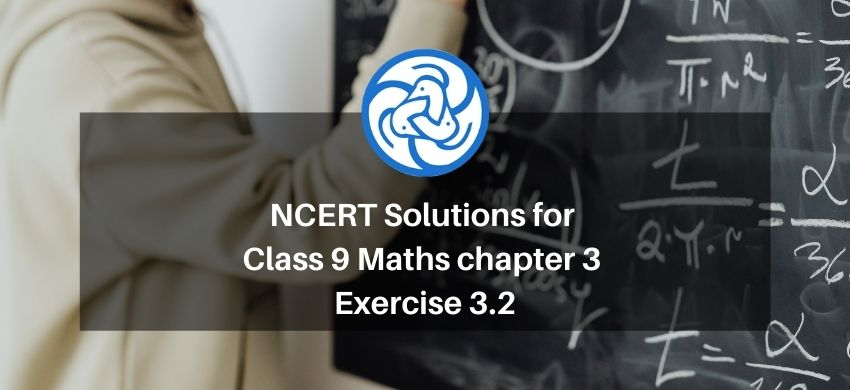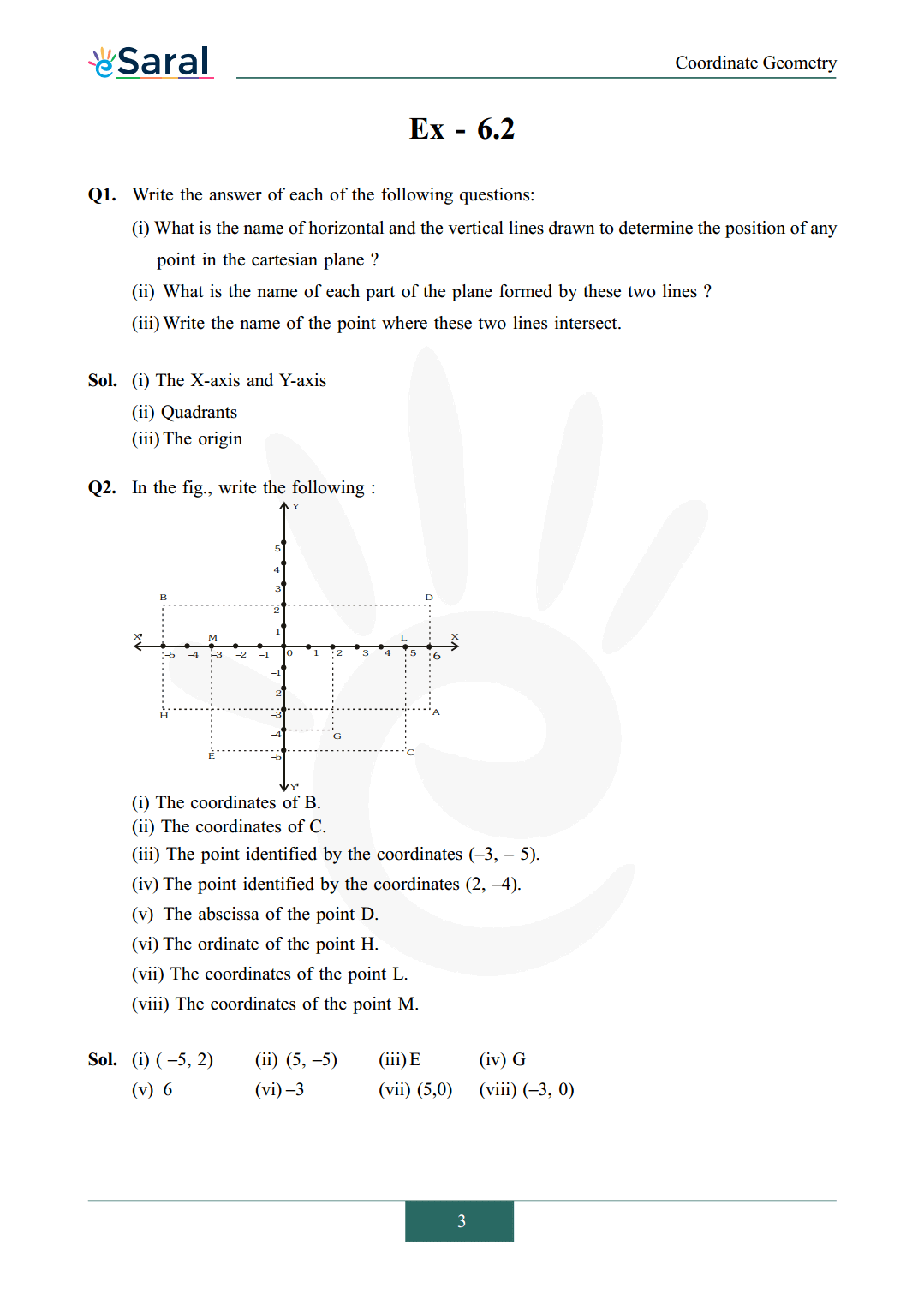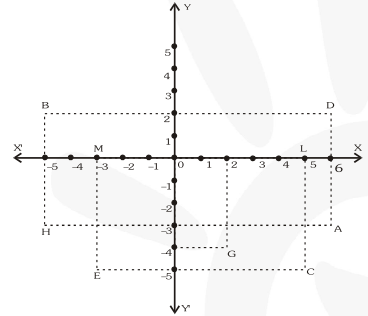# NCERT Solutions for Class 9 Maths chapter 3 Exercise 3.2 - Coordinate Geometry - Free PDF DownloadHey, are you a class 9 Student and Looking for Ways to Download NCERT Solutions for Class 9 Maths chapter 3 Exercise 3.2? If Yes then you are at the right place.

Here we have listed Class 9 maths chapter 3 exercise 3.2 solutions in PDF that is prepared by Kota’s top IITian’s Faculties by keeping Simplicity in mind.

If you want to score high in your class 9 Maths Exam then it is very important for you to have a good knowledge of all the important topics, so to learn and practice those topics you can use eSaral NCERT Solutions.

So, without wasting more time Let’s start.

### Download The PDF of NCERT Solutions for Class 9 Maths chapter 3 Exercise 3.2 "Coordinate Geometry"#### All Questions of Chapter 3 Exercise 3.2

Once you complete the chapter 3 then you can revise Ex. 3.2 by solving following questions

Q1. Write the answer of each of the following questions:
(i) What is the name of horizontal and the vertical lines drawn to determine the position of any point in the cartesian plane?
(ii) What is the name of each part of the plane formed by these two lines?
(iii) Write the name of the point where these two lines intersect.

Q2. In the fig., write the following:(i) The coordinates of B.
(ii) The coordinates of C.
(iii) The point identified by the coordinates $(-3, -5)$.
(iv) The point identified by the coordinates $(2, -4)$.
(v) The abscissa of the point D.
(vi) The ordinate of the point H.
(vii) The coordinates of the point L.
(viii) The coordinates of the point M.

Class 9 Maths Chapter 2 Exercise 2.1 Solutions PDF

Class 9 Maths Chapter 2 Exercise 2.2 Solutions PDF

Class 9 Maths Chapter 2 Exercise 2.3 Solutions PDF

Class 9 Maths Chapter 2 Exercise 2.4 Solutions PDF

Class 9 Maths Chapter 2 Exercise 2.5 Solutions PDF

If you have any Confusion related to NCERT Solutions for Class 9 Maths chapter 3 Exercise 3.2 then feel free to ask in the comments section down below.

To watch Free Learning Videos on Class 9 by Kota’s top Faculties Install the eSaral App